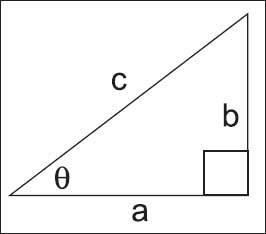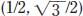NextPrevious

# What is an example of finding a trig function?

One example of finding a trig function—including an illustration—follows: Find the trig functions of the angle θ = 60°. In this case, make a circle with a string extending two units; then measure 60° from the vertex (O). Drop a line perpendicular from the point on the circle to the initial line, creating a 90° angle; that makes the last angle 30°. Because the result is a right triangle, with the values of the hypotenuse (2) and the adjacent leg (1), the Pythagorean theorem (see elsewhere in this chapter) is used to determine the length of the other leg, or a2 + b2 = c2:Trigonometric functions can be used to describe a right triangle.Trigonometric functions of a circle.

Thus, by figuring out the cos (adjacent/hypotenuse) and sin (opposite/hypotenuse) for the angle 60°, Q is at pointon the circle. The other trig functions can be determined using the methods in the questions above:

Close

This is a web preview of the "The Handy Math Answer Book" app. Many features only work on your mobile device. If you like what you see, we hope you will consider buying. Get the App